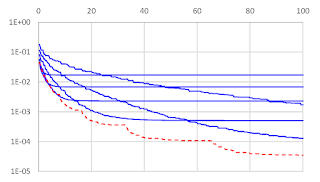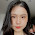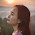## Thursday, May 26, 2016

### The complexity zoo and reductions in optimization

(post by Zeyuan Allen-Zhu and Elad Hazan)

The following dilemma is encountered by many of my friends when teaching basic optimization: which variant/proof of gradient descent should one start with? Of course, one needs to decide on which depth of convex analysis one should dive into, and decide on issues such as "should I define strong-convexity?", "discuss smoothness?", "Nesterov acceleration?", etc.

This is especially acute for courses that do not deal directly with optimization, which is described as a tool for learning or as a basic building block for other algorithms. Some approaches:
• I teach online gradient descent, in the context of online convex optimization, and then derive the offline counterpart. This is non-standard, but permits an extremely simple derivation and gets to the online learning component first.
• Sanjeev Arora teaches basic offline GD for the smooth and strongly-convex case first.
• In OR/optimization courses the smooth (but not strongly-convex) case is many times taught first.
All of these variants have different proofs whose connections are perhaps not immediate. If one wishes to go into more depth, usually in convex optimization courses one covers the full spectrum of different smoothness/strong-convexity/acceleration/stochasticity regimes, each with a separate analysis (a total of 16 possible configurations!)

This year I've tried something different in COS511 @ Princeton, which turns out also to have research significance. We've covered basic GD for well-conditioned functions, i.e. smooth and strongly-convex functions, and then extended these result by reduction to all other cases!
A (simplified) outline of this teaching strategy is given in chapter 2 of this book.

### Classical Strong-Convexity and Smoothness Reductions

Given any optimization algorithm A for the well-conditioned case (i.e., strongly convex and smooth case), we can derive an algorithm for smooth but not strongly functions as follows.

Given a non-strongly convex but smooth objective $f$, define a objective by
$$\text{strong-convexity reduction:} \qquad f_1(x) = f(x) + \epsilon \|x\|^2$$
It is straightforward to see that $f_1$ differs from $f$ by at most $\epsilon$ times a distance factor, and in addition it is $\epsilon$-strongly convex. Thus, one can apply $A$ to minimize $f_1$ and get a solution which is not too far from the optimal solution for $f$ itself.  This simplistic reduction yields an almost optimal rate, up to logarithmic factors.

Similar simplistic assumptions can be derived for (finite-sum forms of) non-smooth by strongly-convex functions (via randomized smoothing or Fenchel duality), and for functions that are neither smooth nor strongly-convex by just applying both reductions simultaneously. Notice that such classes of functions include famous machine learning problems such as SVM, Logistic Regression, SVM, L1-SVM, Lasso, and many others.

### Necessity of Reductions

This is not only a pedagogical question. In fact, very few algorithms apply to the entire spectrum of strong-convexity / smoothness regimes, and thus reductions are very often intrinsically necessary. To name a few examples,
• Variance-reduction methods such as SAG, SAGA and SVRG require the objective to be smooth, and do not work for non-smooth problems like SVM. This is because for loss functions such as hinge loss, no unbiased gradient estimator can achieve a variance that approaches to zero
• Dual methods such as SDCA or APCG require the objective to be strongly convex, and do not directly apply to non-strongly convex problems. This is because for non-strongly convex objectives such as Lasso, their duals are not even be well-defined.
• Primal-dual methods such as SPDC require the objective to be both smooth and SC.

### Optimality and Practicality of Reductions

The folklore strong-convexity and smoothing reductions are suboptimal. Focusing on the strong-convexity reduction for instance:
• It incurs a logarithmic factor log(1/ε) in the running time so leading to slower algorithms than direct methods. For instance, if $f(x)$ is smooth but non-strongly convex, gradient descent minimizes it to an $\epsilon$ accuracy in $O(1/\epsilon)$ iterations; if one uses the reduction instead, the complexity becomes $O(\log(1/\epsilon)/\epsilon)$.
• More importantly, algorithms based on such reductions become biased: the original objective value $f(x)$ does not converge to the global minimum, and if the desired accuracy is changed, one has to change the weight of the regularizer $\|x\|^2$ and restart the algorithm.
These theoretical concerns also translate into running time losses and parameter tuning difficulties in practice. For such reasons, researchers usually make efforts on designing unbiased methods instead.
• For instance, SVRG in theory solves the Lasso problem (with smoothness L) in time $O((nd+\frac{Ld}{\epsilon})\log\frac{1}{\epsilon})$ using reduction. Later, direct and unbiased methods for Lasso are introduced, including SAGA which has running time $O(\frac{nd+Ld}{\epsilon})$, and SVRG++ which has running time $O(nd \log\frac{1}{\epsilon} + \frac{Ld}{\epsilon})$.
One can find academic papers derive various optimization improvements many times for only one of the settings, leaving the other settings desirable. An optimal and unbiased black-box reduction is thus a tool to extend optimization algorithms from one domain to the rest.

### Optimal, Unbiased, and Practical Reductions

In this paper, we give optimal and unbiased reductions. For instance, the new reduction, when applied to SVRG, implies the same running time as SVRG++ up to constants, and is unbiased so converges to the global minimum. Perhaps more surprisingly, these new results imply new theoretical results that were not previously known by direct methods. To name two of such results:
• On Lasso, it gives an accelerated running time $O(nd \log\frac{1}{\epsilon} + \frac{\sqrt{nL}d}{\sqrt{\epsilon}})$ where the best known result was not only an biased algorithm but also slower $O( (nd + \frac{\sqrt{nL}d}{\sqrt{\epsilon}}) \log\frac{1}{\epsilon} )$.
• On SVM with strong convexity $\sigma$, it gives an accelerated running time $O(nd \log\frac{1}{\epsilon} + \frac{\sqrt{n}d}{\sqrt{\sigma \epsilon}})$ where the best known result was not only an biased algorithm but also slower $O( (nd + \frac{\sqrt{n}d}{\sqrt{\sigma \epsilon}}) \log\frac{1}{\epsilon} )$.
These reductions are surprisingly simple. In the language of strong-convexity reduction, the new algorithm starts with a regularizer $\lambda \|x\|^2$ of some large weight $\lambda$, and then keeps halving it throughout the convergence. Here, the time to decrease $\lambda$ can be either decided by theory or by practice (such as by computing duality gap).

A figure to demonstrate the practical performance of our new reduction (red dotted curve) as compared to the classical biased reduction (blue curves, with different regularizer weights) are presented in the figure below.As a final word - if you were every debating whether to post your paper on ArXiV, yet another example of how quickly it helps research propagate:  only a few weeks after our paper was made available online, Woodworth and Srebro have already made use of our reductions in their new paper.

1.I think that this is really complicated to teach basic optimization: which variant/proof of gradient descent should one start with? This is especially acute for online translation services reviews courses that do not deal directly with optimization, which is described as a tool for learning or as a basic building block for other algorithms. One can find academic papers derive various optimization improvements many times for only one of the settings, leaving the other settings desirable.

2.I want to we appreciate you this passion you cash in on throughout establishing the next few paragraphs.Naturally I’ll give you a link in your internet blog. Thanks for sharing.
best autoketing, Shopify free shipping bar app, shipping bar shopify

3.This is a great inspiring article. I have to thank you for ones time for this especially astonishing read! You can do something much better but i still say this perfect.Keep trying for the best.
facebook live chat help, facebook messenger online, autoketing.com

4.email with love online
apps autoketing
email with love free
Hey there! Do you know if they make any plugins to safeguard against hackers?
I’m kinda paranoid about losing everything I’ve worked hard on. Any suggestions?

5.I accidentally read this and it really made me think. I will have to work hard to make my website useful and more popular. Everyone please refer to offline !!! thank you very much
currency converter master, currency converter box by autoketing, best autoketing app

6.iogames for school
friv land now
abcya games for boy
This pass practice is commonly costly as professional web developers can warfare you each and every share of one from a hundred bucks to thousands of dollars, depending not in the isolate away away off from how perplexing you nonappearance your website to be

7.I want to read your articles.Thanks to your sharing, I can enrich my knowledge. thank you for the wonderful sharing. They are useful pieces of advice. abcya3 Games for boys juegos motox3m3 gogy for school

8.I'm a decent implied for all articles or blog entries, That I emphatically relished, We'd to a great degree pick much more records identified with the, because of the reality it is in reality okay
The story of cinderella Games to play
Crime city 3d 2 Games online
Top burger Games for girls

9.When I have spare time, I often read your posts. They help me so much. Thanks a million. I am waiting new posts. I usually wait for your pieces of writing.
play Battle for the Galaxy Break The Cup For Free Toon Cup 2017

10.Your posts are the flower of the flock.I decided to add your blog to my bookmarks so I can return to it at a later date.
online free game Blocky Craft Police Squad Mommy Elsa Baby Caring play for free best game Tomb Runner

11.Wow, cool post. I'd like to write like this too - taking time and real hard work to make a great article... but I put things off too much and never seem to get started. Thanks though.
Games xmas magic tiles
Truck physics free
Gunmaster onslaught games for kid

12.Elsa breakup drama best online game
Fire truck crazy race games for boy
Unblock the ball games online
Great info! I recently came across your blog and have been reading along. I thought I would leave my first comment. I don’t know what to say except that I have

13.Good post, it is well worth letting people take the time to track it.
Music Line 2 Christmas interesting games, Hit The Glow free online juegos, Happy Glass good games for kids